﻿ Excel for .NET for WinForms: Creating Excel Documents on the Fly# Excel for .NET for WinForms: Creating Excel Documents on the Fly

ComponentOne Studio Excel for .NET for WinForms is easy to use, allowing us to use a single command to load or save a workbook from the .NET code. Workbooks, which can contain multiple sheets, can be directly read to and written from memory streams too, so we don't have to use temporary files. We don't even need to have Microsoft Excel installed! Let's say, we have regional data about the sales of some stationery products. The requirement is to analyze thedata and present the final document to the area Sales Managers. The idea here is to create and format a professional-looking Excel file out of the existing data. With the built-in features that Excel for .NET offers, achieving such requirements becomes easy.

### Create an Excel File and Add Sheets to it

First and foremost, we need to create an Excel file and add sheets to it. For creating the Excel workbook, create an object of C1XLBook class (which represents an Excel Workbook) in your application. It's a Non-GUI control which would not be shown on the Form; it is used to generate the Excel books. Now is the time to add sheets to the Excel book using XLSheet class and then add it to the XLSheetCollection of C1XLBook.

``````
//Creating an Excel Book
C1XLBook xlbook1 = new C1XLBook();
xlbook1.Sheets.Clear();
XLSheet sheet1 = xlbook1.Sheets.Add("New Sheet 1");

``````

This code snippet will generate a blank Excel Workbook, which can be used for storing data.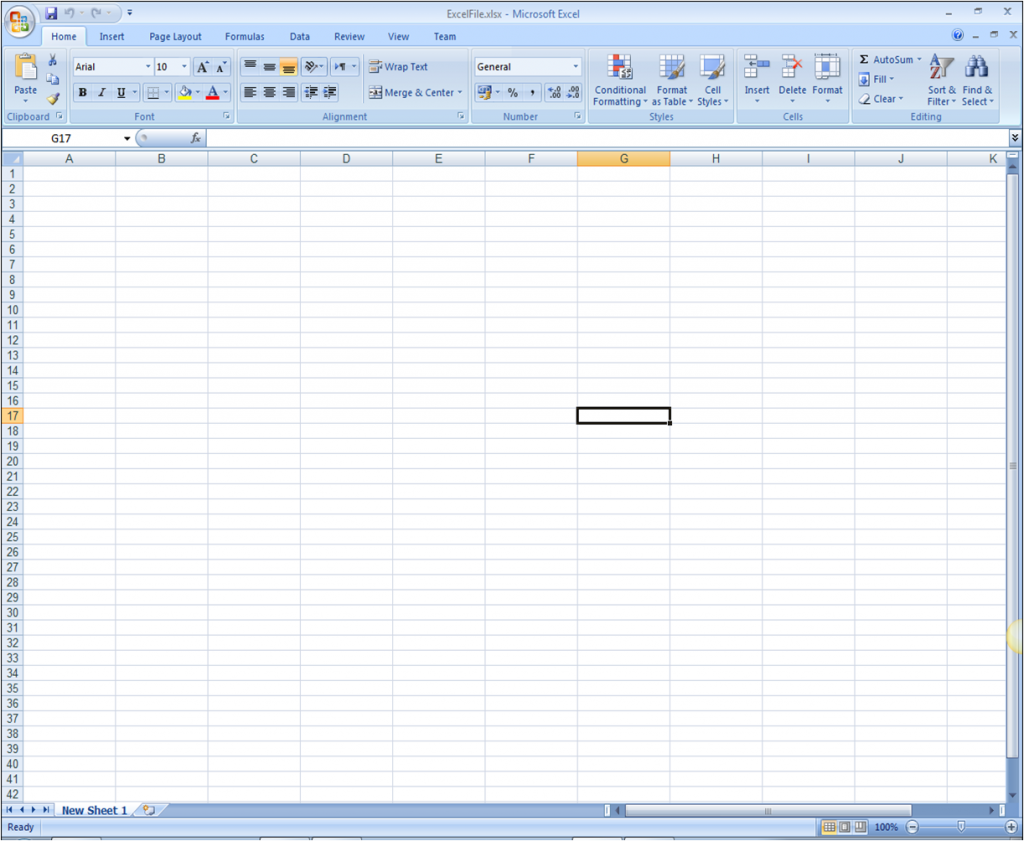### Add the Content in the Excel Sheet

To add the regional sales data in the new sheet, we can use the indexer in the XLSheet object. It represents each cell in the XLSheet and using the Value property of that cell, the content can be added.

``````

sheet1[0, 0].Value = "OrderDate";
sheet1[1, 0].Value = "23-01-2014";
sheet1[2, 0].Value = "09-02-2014";
sheet1[3, 0].Value = "26-02-2014";
sheet1[4, 0].Value = "15-03-2014";
sheet1[5, 0].Value = "4-1-2014";
sheet1[6, 0].Value = "18-4-2014";
sheet1[7, 0].Value = "5-5-2014";
sheet1[8, 0].Value = "22-5-2014";
sheet1[9, 0].Value = "6-8-2014";
sheet1[10, 0].Value = "1-6-2014";
sheet1[0, 1].Value = "Region";
sheet1[1, 1].Value = "East";
sheet1[2, 1].Value = "Central";
sheet1[3, 1].Value = "Central";
sheet1[4, 1].Value = "Central";
sheet1[5, 1].Value = "West";
sheet1[6, 1].Value = "East";
sheet1[7, 1].Value = "Central";
sheet1[8, 1].Value = "East";
sheet1[9, 1].Value = "East";
sheet1[10, 1].Value = "West";

``````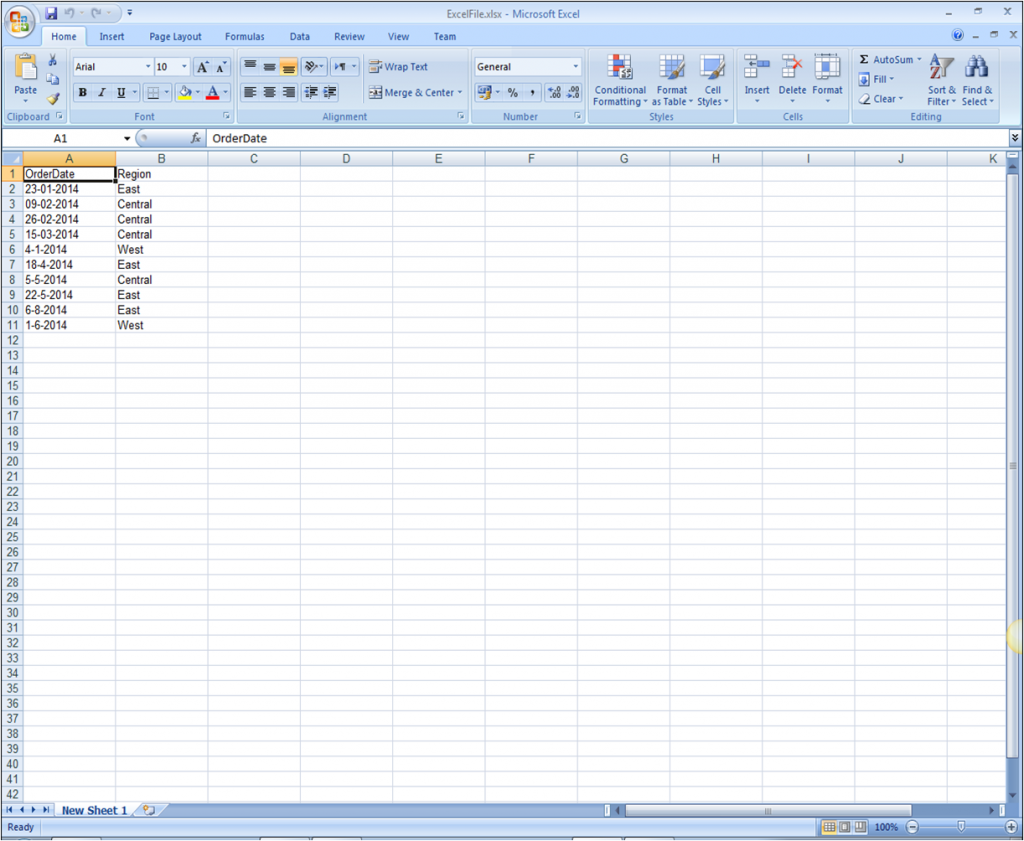### Styling the Cell Content of the Excel Sheet

Styling and formatting is very important when it comes to analyze and present data. Excel for .NET provides a class XLStyle to define and modify the appearance of the cell, row and column. It includes the style elements such as Font, alignment, colors and format.

``````
XLStyle style_all = new XLStyle(xlbook1);
style_all.AlignHorz = XLAlignHorzEnum.Center;

for (int j = 0; j < 7; j++)
{
sheet1.Columns[j].Style = style_all;
}

style_header.Font = new Font("Tahoma", 11, FontStyle.Bold | FontStyle.Underline);

for (int i = 0; i < 7; i++)
{
// Apply styles to the content.
}

// Styles to Rows and Columns
XLStyle style_col = new XLStyle(xlbook1);
style_col.Font = new System.Drawing.Font("Arial", 12, FontStyle.Bold);
style_col.ForeColor = Color.Green;
style_col.AlignHorz = XLAlignHorzEnum.Center;

XLColumn col = sheet1.Columns;
col.Style = style_col;

``````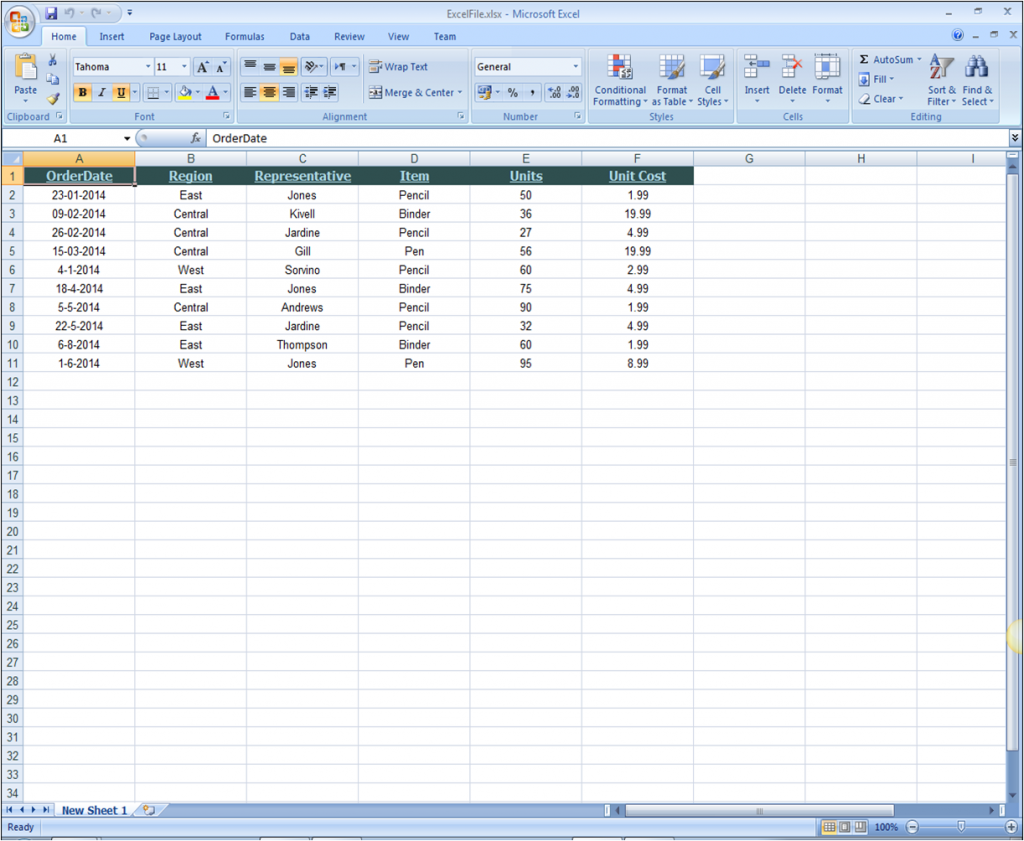### Applying Formula to the Sheet

Since we're presenting the regional sales data, we need to perform certain calculations in order to summarize the document. In order to fulfill such calculation-related requirements, Excel for .NET provides full support for binary parsing and creating formulas using the Formula property of an XLCell. Same formulas, like those in MS Excel, can be used on the cells of the XLSheet.

``````
//Formula to calculate the Total units sold and Total order cost
sheet1[11, 3].Value = "Total Units";
sheet1[11, 4].Formula = "Sum(E2:E11)";

sheet1[11, 5].Value = "Total Order Cost";
sheet1[11, 6].Formula = "Sum(G2:G11)";

//Formula to calculate the total cost
for (int i = 2; i < 12; i++)
{
sheet1[i - 1, 6].Formula = "F" + i.ToString() + "*" + "E" + i.ToString();
}

XLStyle style_total = new XLStyle(xlbook1);
style\_total.Font = new System.Drawing.Font(style\_total.Font.FontFamily, 12, FontStyle.Bold);
style_total.SetBorderStyle(XLLineStyleEnum.Medium);
style_total.AlignHorz = XLAlignHorzEnum.Center;

sheet1[11, 3].Style = style_total;
sheet1[11, 4].Style = style_total;
sheet1[11, 5].Style = style_total;
sheet1[11, 6].Style = style_total;

``````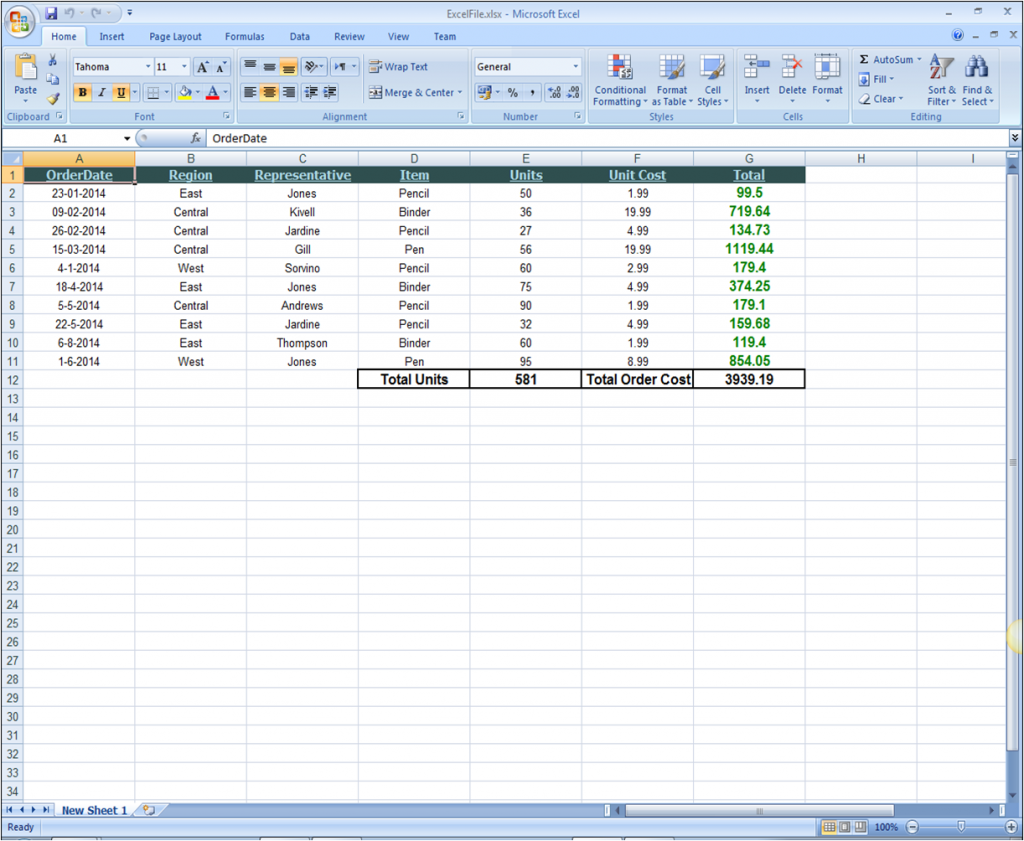### Adding MS Excel-like Comment in the Sheet

``````

``````

The comment in the document will be notified by a triangle in the top right corner of the cell and it can be viewed by hovering the mouse over the cell.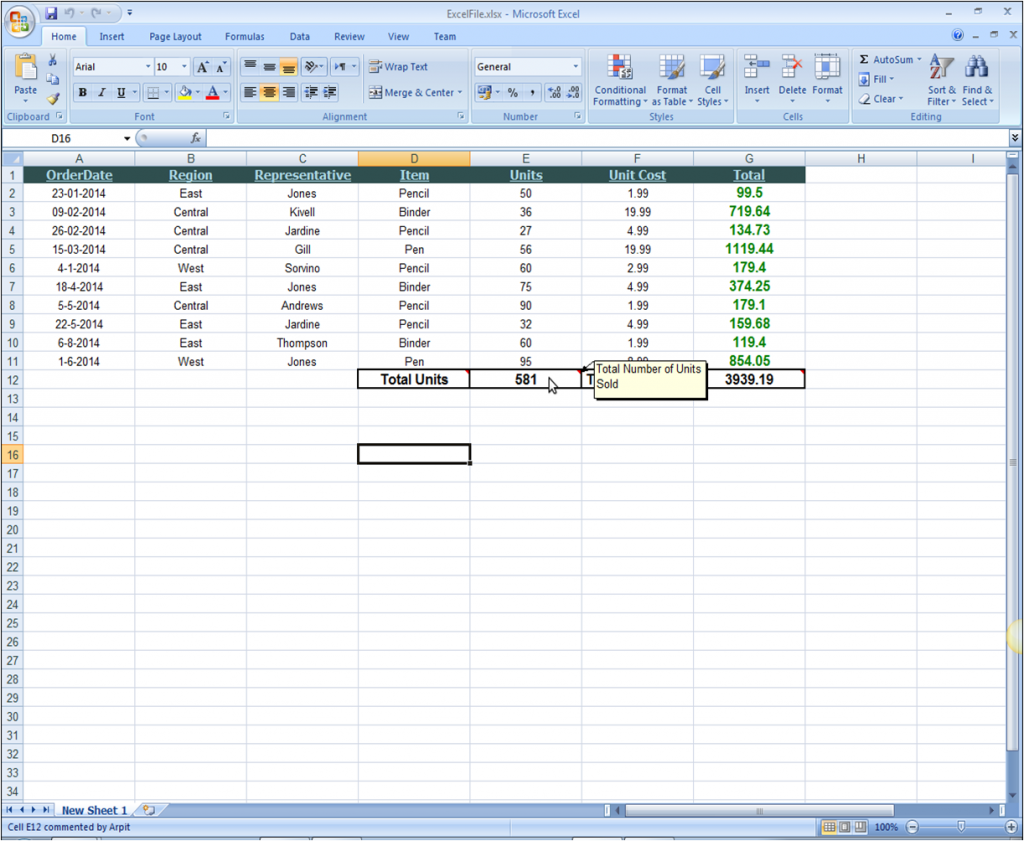### Merging Cells of the Sheet

Excel for .NET contains a property MergedCells which can be used to create the merged ranges in the Excel file. The cells to be merged together are added into the MergedCells XLCellRangeCollection.

``````
//Select range to Merge
XLCellRange merge_range1 = new C1.C1Excel.XLCellRange(2, 4, 1, 1);
XLCellRange merge_range2 = new C1.C1Excel.XLCellRange(8, 9, 1, 1);
XLCellRange merge_range3 = new C1.C1Excel.XLCellRange(7, 8, 3, 3);

//Merge Cells

``````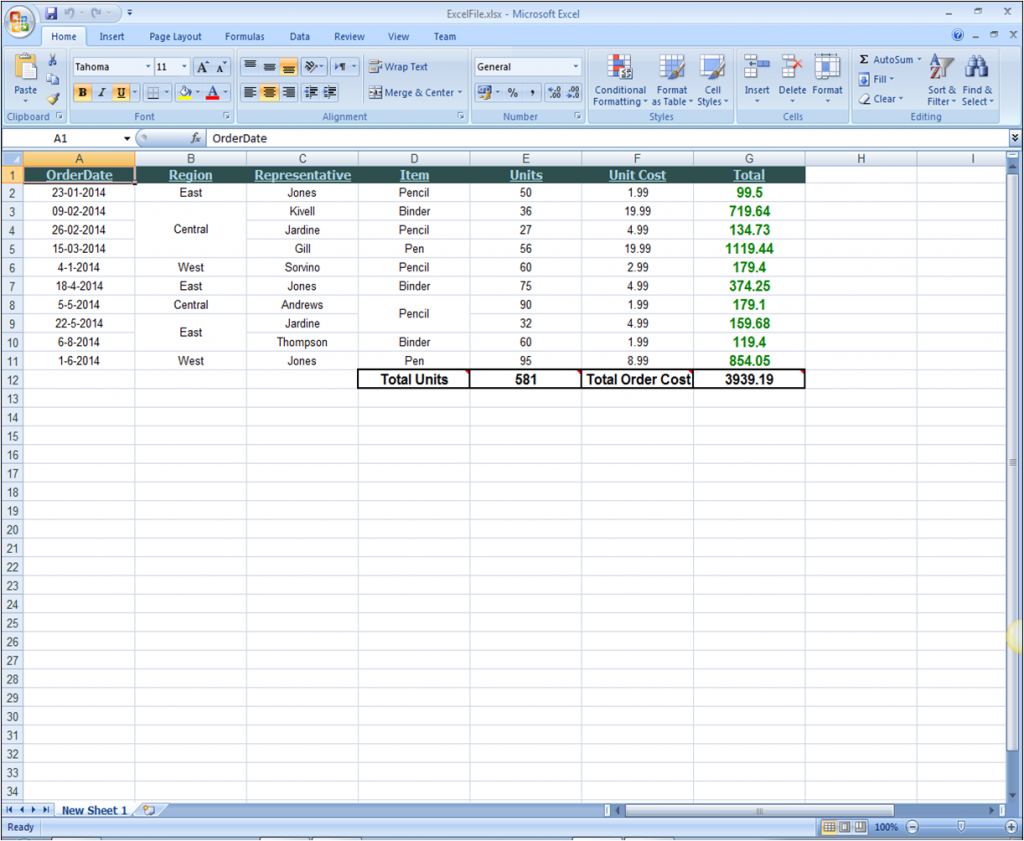### Adding Images in the Excel Workbook

Excel for .NET supports adding of images to a sheet or a cell. Images from the system or streams can be accessed using the Image class and can be directly assigned to the Value property of the cell.

``````
Image img = Image.FromFile("..\\\..\\\vbstationers.jpg");
sheet1[13, 2].Value = img;

``````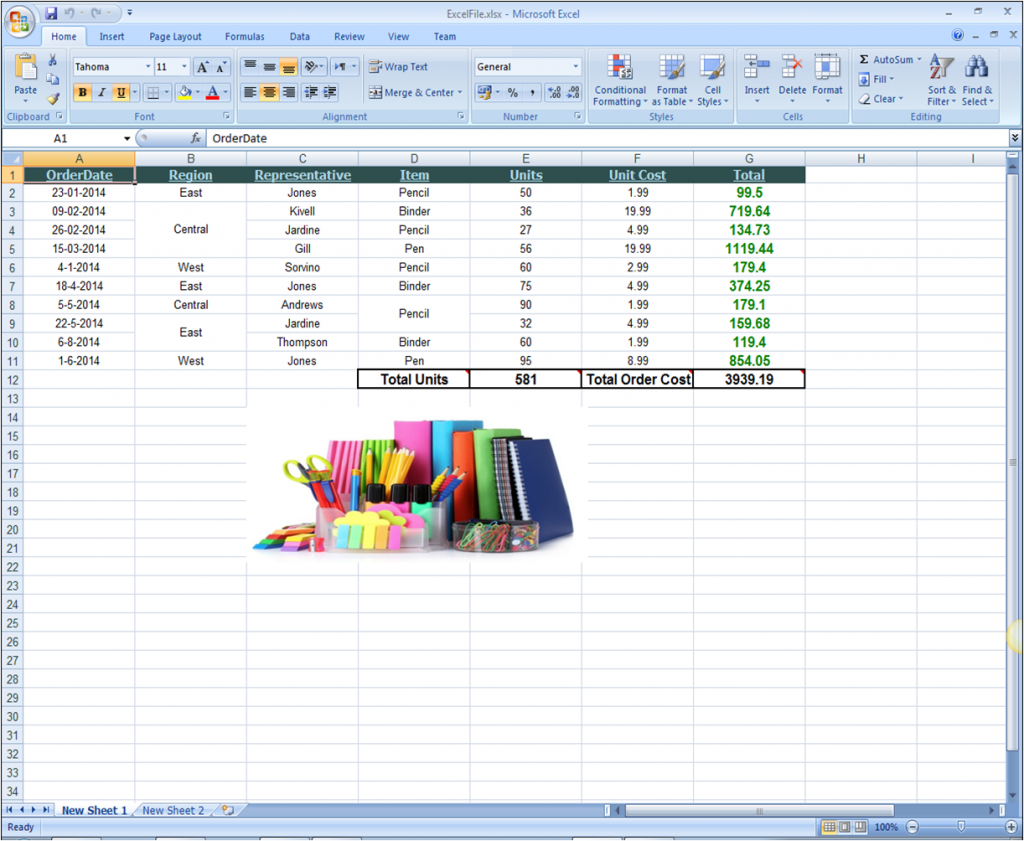### Adding PageBreaks and Creating PrinterSettings

Page break is a marker in an electronic document that tells the document interpreter that the content which follows is part of a new page. In ComponentOne Excel for .NET, page breaks can be easily added in rows and columns of a sheet to divide the document in different pages by using the PageBreak property of Rows and Columns. Moreover, using XLPrintSettings class of C1Excel, printer settings for the document can also be customized using code only.

``````
sheet1.Rows.PageBreak = true;
sheet1.Columns.PageBreak = true;

// Print Settings for the document
XLPrintSettings settings = sheet1.PrintSettings;
settings.BlackAndWhite = true;
settings.Copies = 2;
settings.Footer = "VC Stationers";
settings.AlignWithMargins = true;
settings.MarginTop = 1;
settings.MarginBottom = 1;
settings.MarginLeft = 0;
settings.MarginRight = 0;
settings.Landscape = true;
settings.CenterHorizontal = true;

``````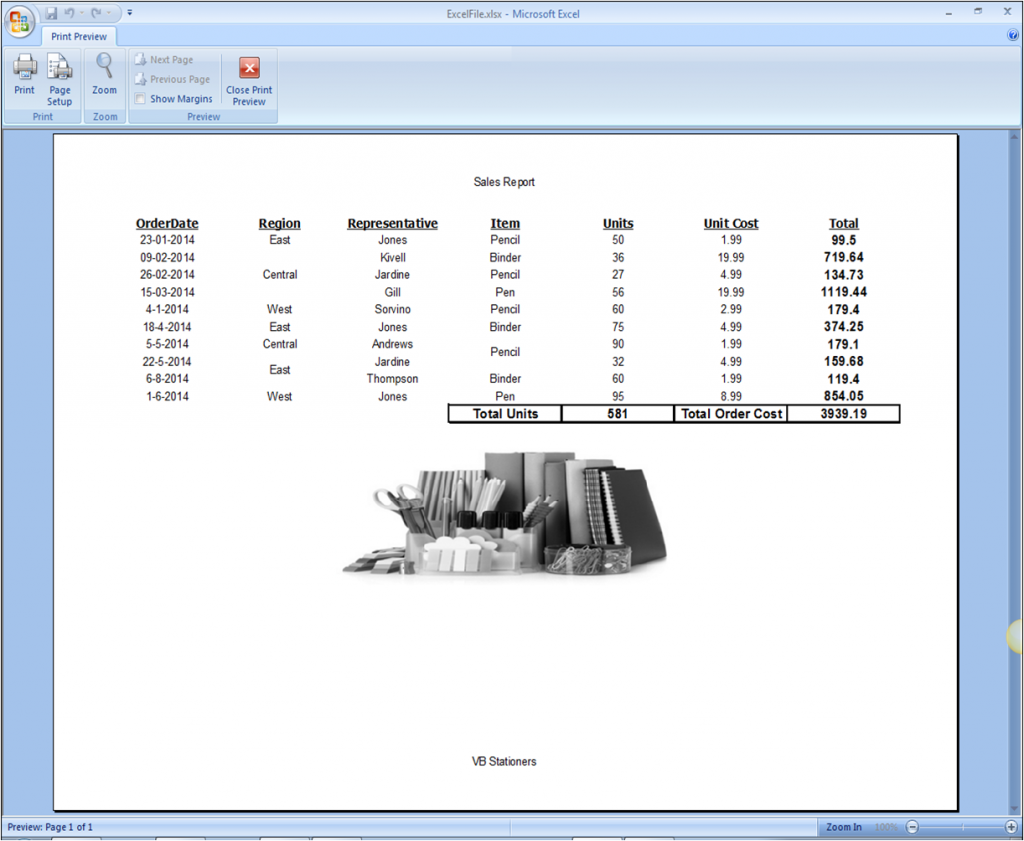### Resultant Excel Workbook

After summing up all the objects and code snippets discussed above, the complete Excel Workbook will look like the following image.### Working SamplesGrapeCity

GrapeCity Developer Tools